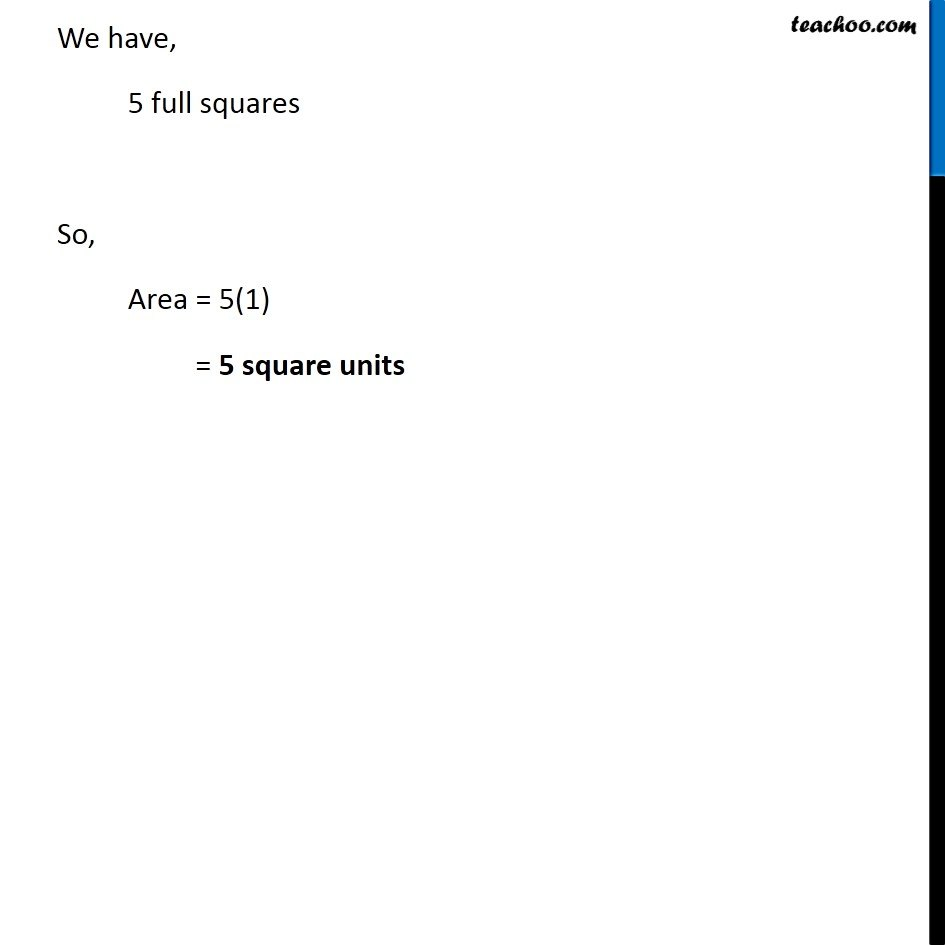1. Chapter 10 Class 6 Mensuration
2. Serial order wise
3. Ex 10.2

Transcript

Ex 10.2, 1 (h) Find the areas of the following figures by counting square: Covered Area Number Area 1 Fully filled squares 5 1 × 5 = 5 2 Half filled squares 0 0 × 1/2 = 0 3 More than Half filled squares 0 0 × 1 = 0 4 Less than half filled squares 0 0 × 0 = 0 We have, 5 full squares So, Area = 5(1) = 5 square units

Ex 10.2

Chapter 10 Class 6 Mensuration
Serial order wise